# mathematics## Maths solutions,ssc 10th class real numbers chapter 1,exercise 1.1, 1.2, 1.3, 1.4 and 1.5, ap and ts

Maths solutions for ssc or 10th class real numbers chapter 1 (ap and ts ) You can see the solutions for maths 10th class real numbers for some problems ( text book ). These solutions are very easy to understand. Andhra pradesh and telangana 10 th class mathematics solutions for real numbers. SSC maths solutions …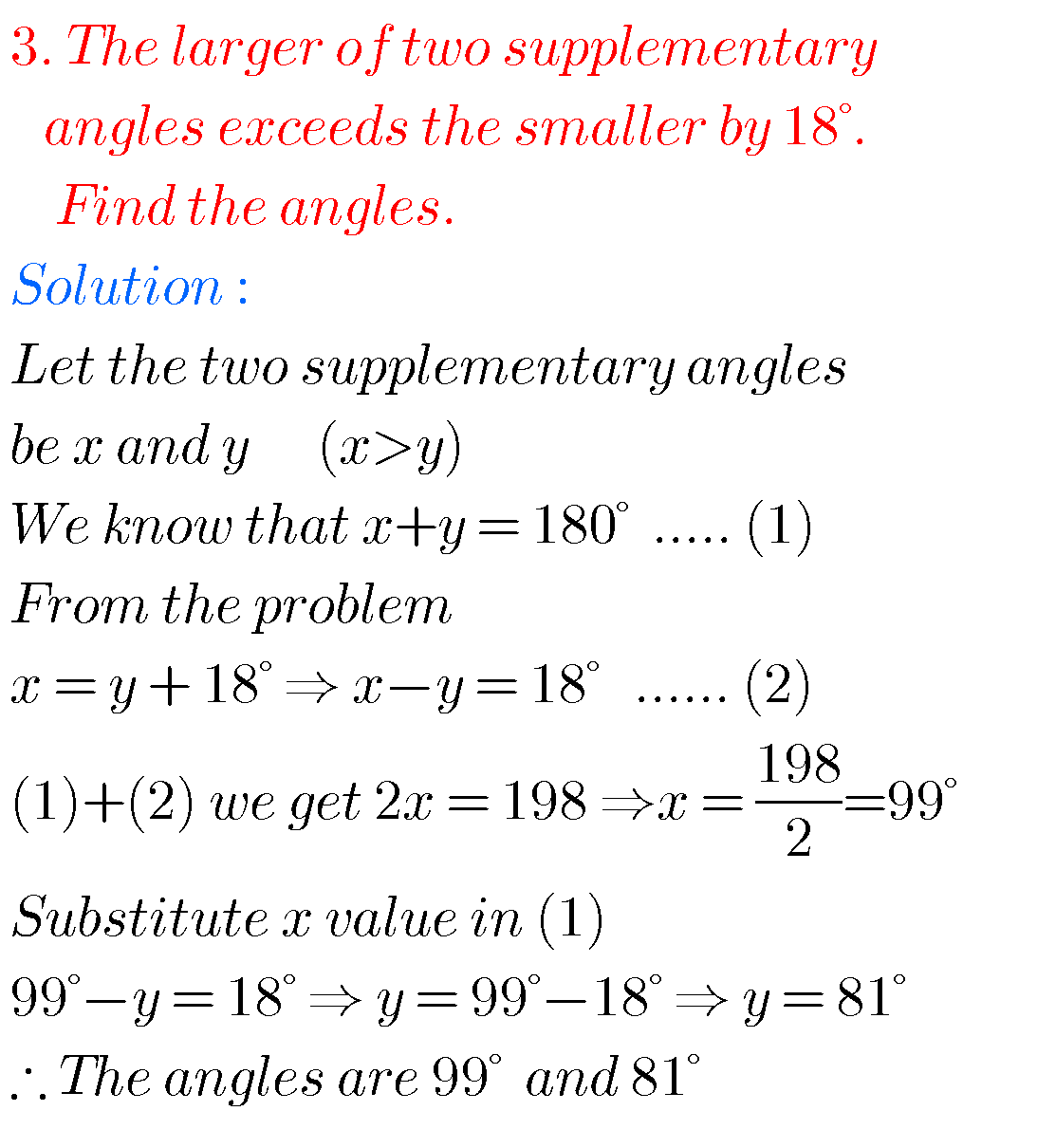## SSC maths solutions for Pair of linear equations in two variables class 10

SSC maths solutions for pair of Linear Equations in Two Variables SSC mathematics class 10 pair of linear equations in two variables solutions for text book exercises. These solutions are very easy to understand. First study the text book well. Exercise 4.1, exercise 4.2 and exercise 4.3  solutions are given.  You can also see the …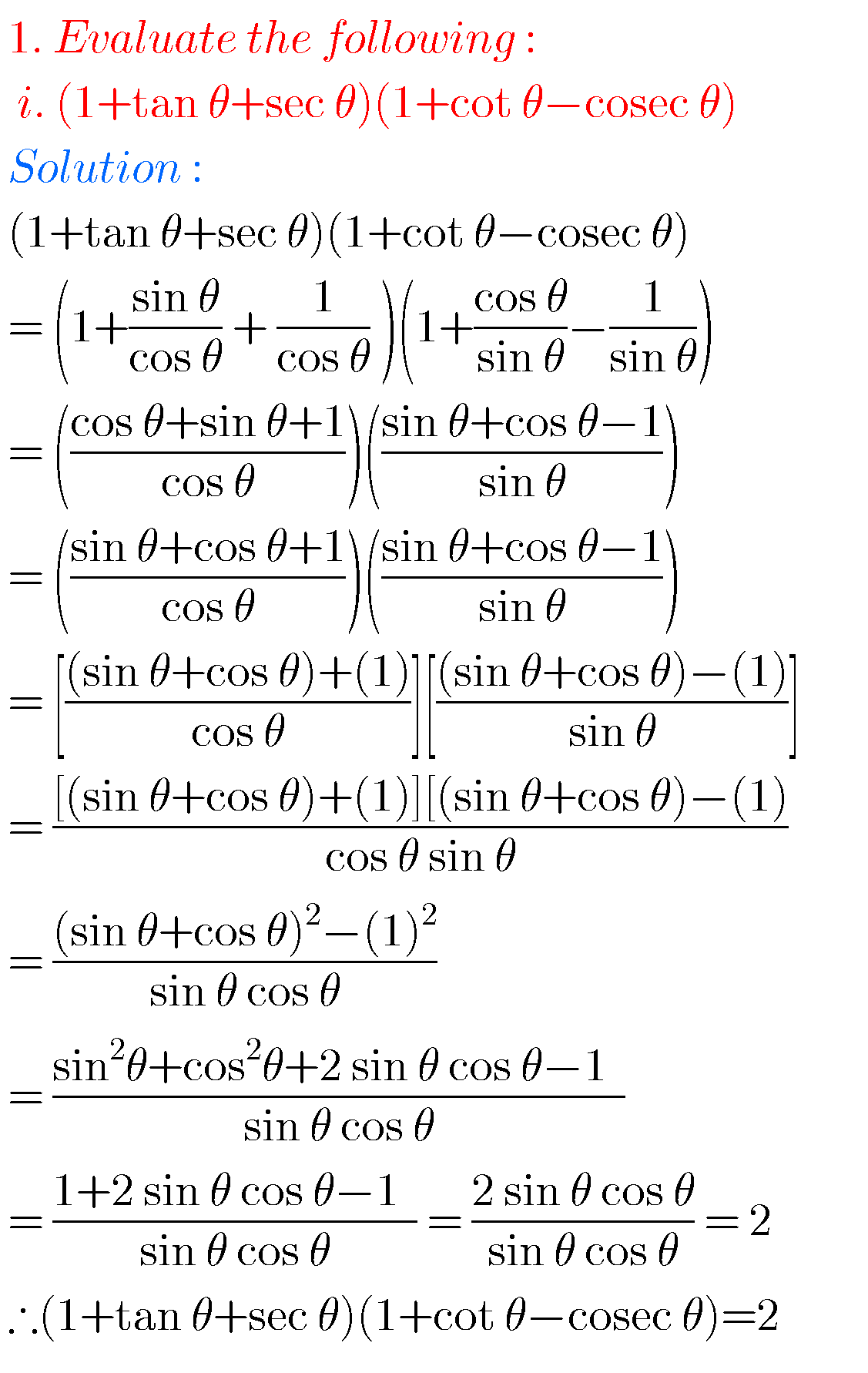## SSC mathematics Trigonometry solutions class 10

Trigonometry solutions class 10 maths ssc Tenth class mathematics chapter 11 trigonometry exercises 11.1, 11.2, 11.3, 11.4, solutions are given. These solutions are very easy to understand. First study the chapter 11 very well. Practice example problems and solutions. Observe the solutions and try them in your own method. Class 10 maths trigonometry solutions You …## Maths solutions for applications of trigonometry class 10 ssc

Maths solutions for applications of trigonometry class 10 ssc Tenth class mathematics chapter 12 exercises 12.1 and 12.2 solutions are given.  These solutions are very easy to understand. First study the chapter 12 well for many times. Observe the example probems and solutions well. Try them in your own method. Next solve the exercise problems. …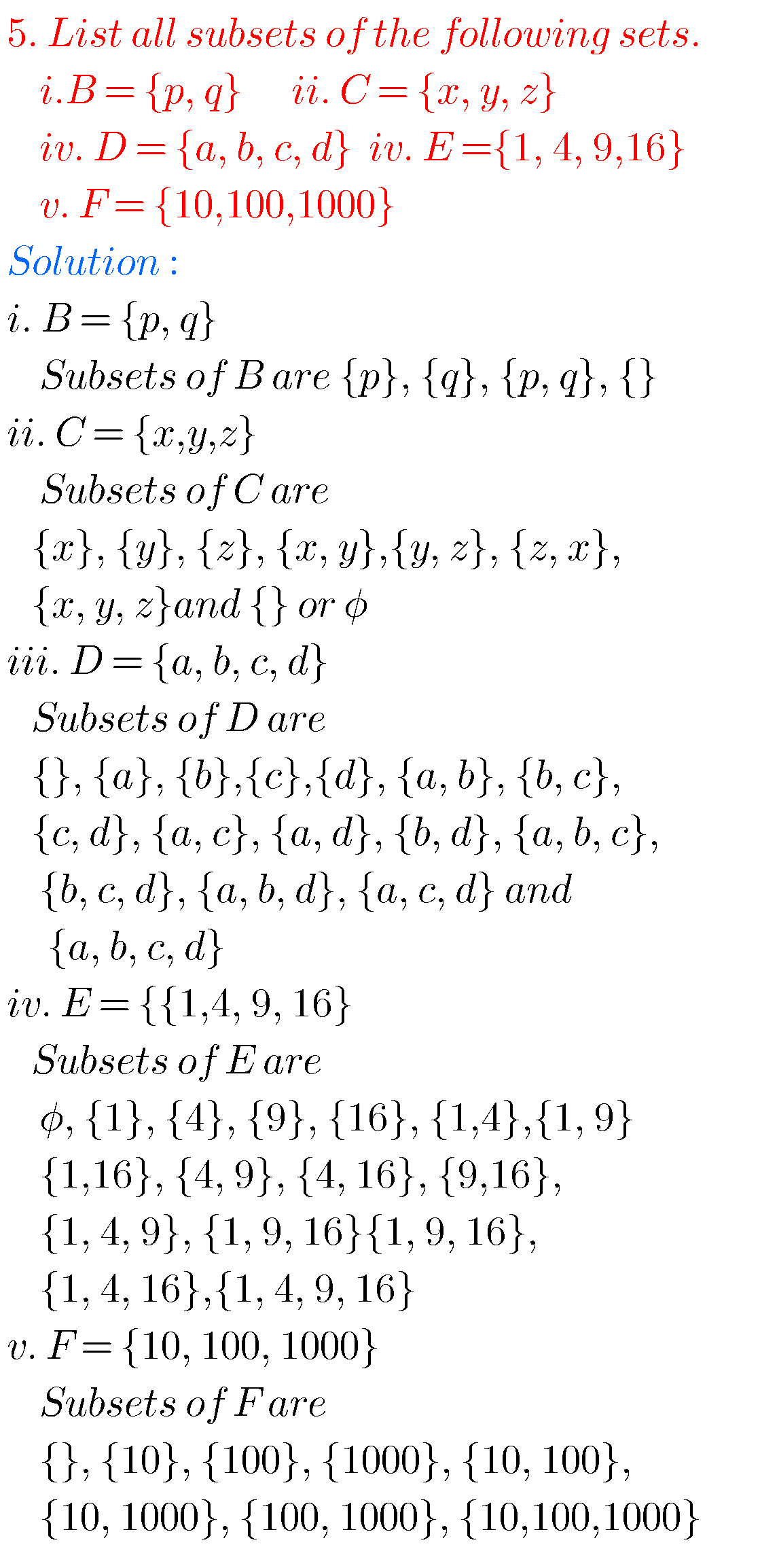## Tenth maths guide for sets,ssc mathematics solutions for chapter 2 sets(class 10),exercise 2.1, 2.2, 2.3, 2.4

Tength maths guide for sets Tenth class maths solutions for sets.These solutions are very easy to understand. Mathematics 10th class Andhra predesh and telangana. Solutions are given for text book problems. SSC maths solutions for tenth class sets questions. Observe the solutions and try them in your own method. Inter maths 1a solutions You can …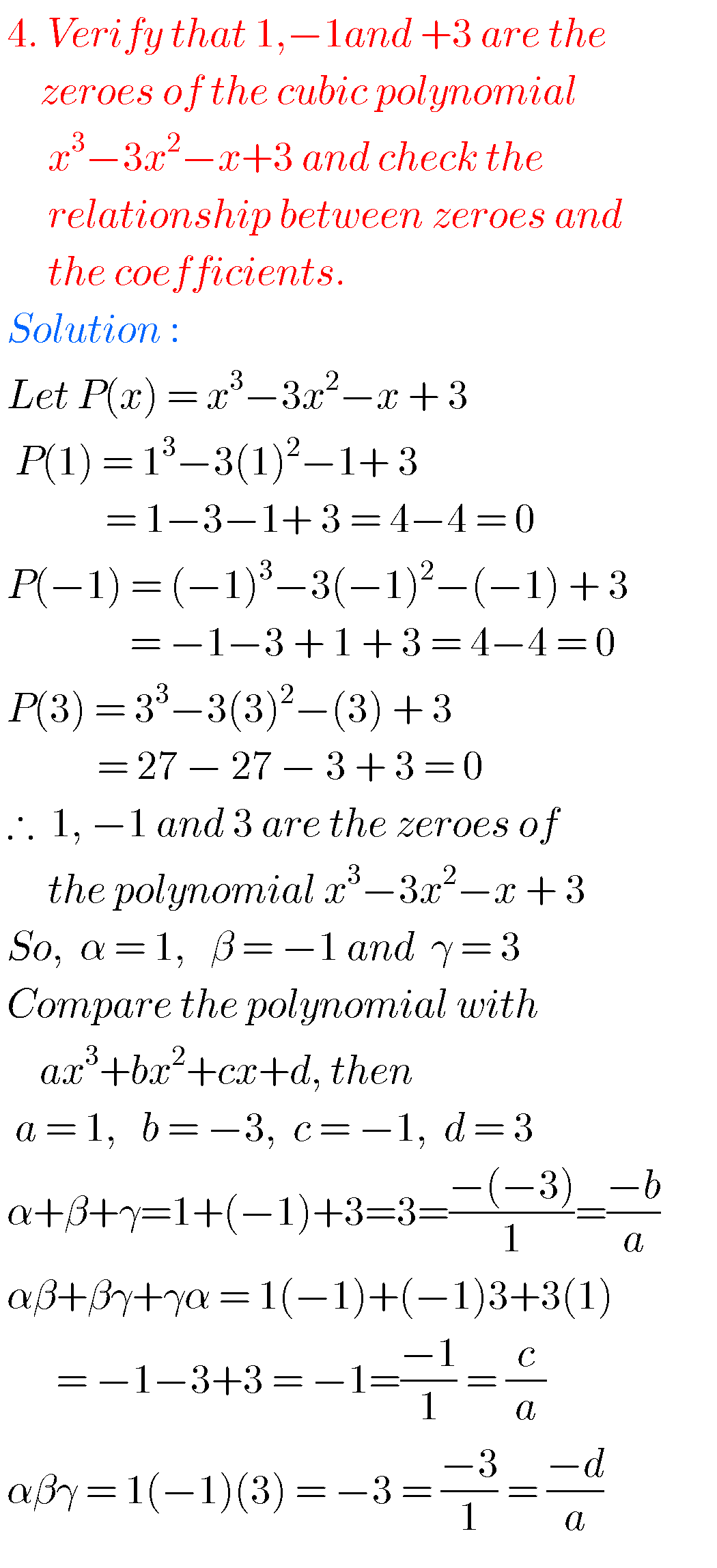## Tenth maths guide for polynomials,exercise 3.1, 3.2, 3.3, 3.4,solutions for class 10 maths chapter 3

Tenth maths guide for polynomials, exercise 3.1, 3.2, 3.3, 3.4. Tenth class mathematics solutions for text book problems. These are very easy to understand. Maths solutions for 10th class Andhra pradesh and Telangana. SSC maths solutions for chapter 3 polynomials. You can also see the solutions for 1. Real numbers 2. Sets 3. Pair of linear equations in …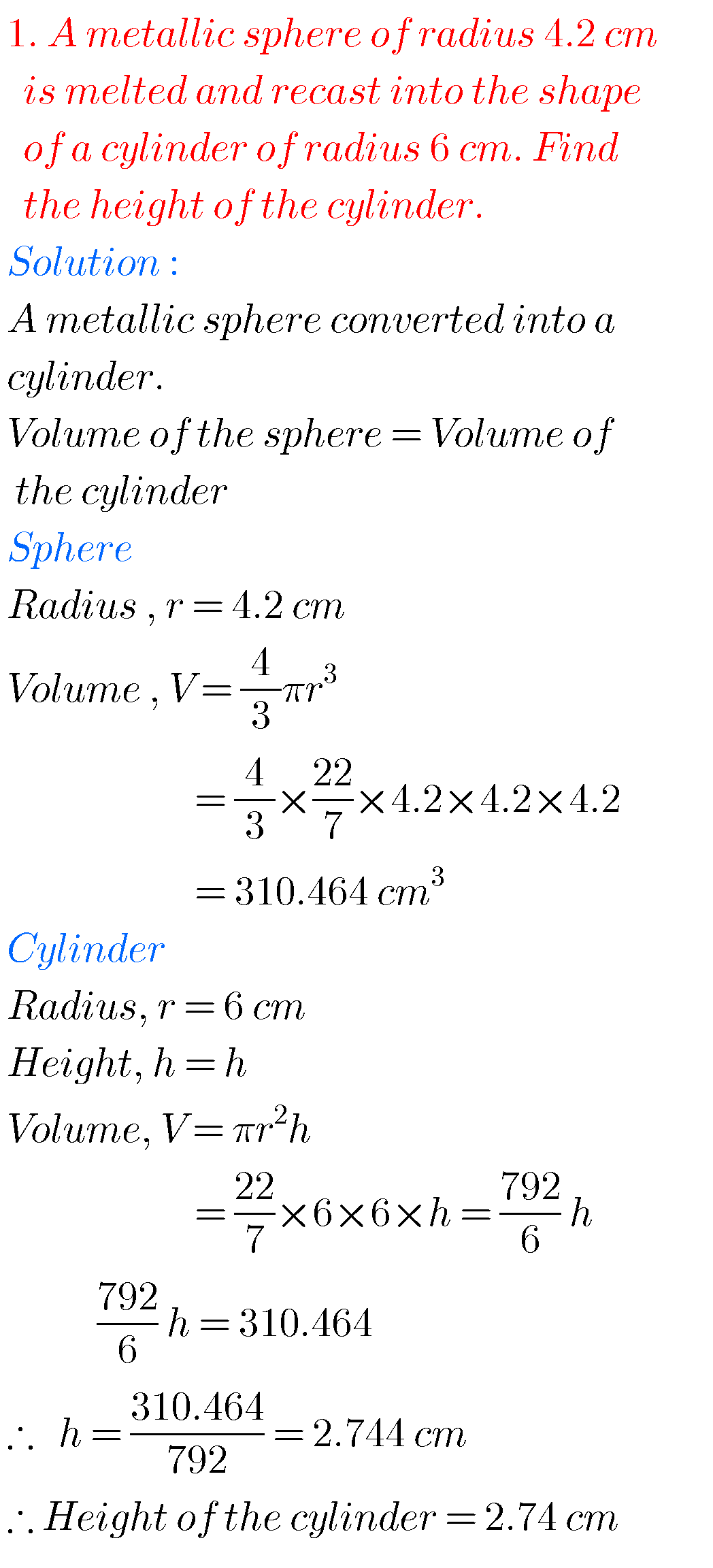## Mensuration solutions class 10 maths ssc

Mensuration solutions class 10 maths ssc SSC maths mensurations solutions for class 10 exercise 10.1, 10.2, 10.3 and 10.4 are given. Study the text book very well. Observe the example problems and solutions. The given solutions are very easy to understand. Observe the solutions and try them in your own method. You can see the …## SSC solutions for maths Progressions class 10

SSC solutions for maths Progressions class 10 Mathematics class 10 SSC Progressions text book problems with solutions are given. These are very easy to understand. First you study the text book very well. Observe the example problems and solutions. Observe the solutions and try them in your own method.  You can also see the solutions …## Trigonometric ratios upto transformations,intermediate first year 1A problems with solutions

TRIGONOMETRIC RATIOS UPTO TRANSFORMATIONS, 2 Mathematics Intermediate 1st year mathematics 1A and 1B solutions for some problems. These solutions are very easy to understand. Inter first year 1A : Functions, mathematical induction, addition of vectors, trigonometric ratios upto transformations, trigonometric equations, hyperbolic functions, inverse trigonometric functions and properties of triangles. Inter first year 1B : Locus, transformation …## Properties of triangles, Intermediate mathematics first year problems with solutions,solutions of properties of triangles, junior inter trigonometry

PROPERTIES OF TRIANGLES, INTERMEDIATE MATHEMATICS FIRST YEAR PROBLEMS WITH SOLUTIONS Intermediate mathematics first year 1A and 1B some chapters. The solutions are very easy to understand. Inter first year 1A : Functions, mathematical induction, functions, addition of vectors, trigonometric ratios upto transformations, trigonometric equations, hyperbolic functions, inverse trigonometric functions and properties of triangles. Inter first year …